## Indicators

•Cited by SciELO
•Access statistics

•Cited by Google
•Similars in SciELO
•Similars in Google

## Print version ISSN 2248-6046

### Finanz. polit. econ. vol.7 no.1 Bogotá Jan./June 2015

#### https://doi.org/10.14718/revfinanzpolitecon.2015.7.1.6

ARTÍCULO DE REFLEXIÓN

DOI: http://dx.doi.org/10.14718/revfinanzpolitecon.2015.7.1.6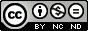EX-POST EQUITY RISK PREMIUMS AND ECONOMIC CYCLES IN COLOMBIA: AN EMPIRICAL RESEARCH USING KALMAN AND HODRICK-PRESCOTT FILTERS

LAS PRIMAS DE RIESGO DE RENTA VARIABLE EX POST Y LOS CICLOS ECONÓMICOS EN COLOMBIA: UNA INVESTIGACIÓN EMPÍRICA UTILIZANDO LOS FILTROS DE KALMAN Y HODRICK-PRESCOTT

OS PRÊMIOS DE RISCO DE VENDA VARIÁVEL EX POST E OS CICLOS ECONÔMICOS NA COLÔMBIA: UMA PESQUISA EMPÍRICA A PARTIR DOS FILTROS DE KALMAN E HODRICK-PRESCOTT

ANDRÉS MAURICIO GÓMEZ SÁNCHEZ a
JOSÉ GABRIEL ASTAIZA GÓMEZ b

a Magíster en Economía. Especialista en Gerencia de Proyectos. Economista. Docente titular e investigador de la Facultad de Ciencias Contables, Económicas y Administrativas de la Universidad del Cauca, Popayán, Colombia. Dirección de correspondencia: carrera 2, calle 15N, esquina, Popayán, Colombia. Correo electrónico: amgomez@unicauca.edu.co
b Magíster en Administración Financiera y especialista en Finanzas. Economista. Docente catedrático de la Universidad del Cauca, Facultad de Ciencias Contables, Económicas y Administrativas. Dirección de correspondencia: carrera 2, calle 15N, esquina, Popayán, Colombia. Correo electrónico: jgastaiza@unicauca.edu.co

Recibido: 3 de julio de 2014. Concepto de evaluación: 23 de noviembre de 2014. Aprobado: 27 de noviembre de 2014

ABSTRACT

This article investigates the relationship between ex-post Equity Risk Premium (ERP) on the Colombian stock market and the economic cycles observed in the country using methodologies based on the Hodrick-Prescott and Kalman filters. Accordingly, a short-term econometric model is put forward for each filter, incorporating monthly data from 2008 to 2014. This reveals better adjustments in the case of the Kalman filter. Furthermore, the models show that the relationship between variables that reflect economic activity and ERP are counter-cyclical but not simultaneous, with a lag of up to two periods.

Keywords: risk premium, economic activity, economic cycle, Kalman filter, Hodrick-Prescott filter, Colombian stock market.

JEL: D91, E21, E32, E44, G11, G12, G14

RESUMEN

Este artículo pretende indagar por la relación existente entre la prima por riesgo ex post (ERP) del mercado accionario colombiano y los ciclos económicos observados para este país, a través de las metodologías del filtro mecánico de Hodrick-Prescott y el filtro de Kalman. Así, se plantea un modelo econométrico a corto plazo para cada uno de los filtros, con información mensual desde 2008 a 2014, lo que permite evidenciar mejores ajustes en el caso Kalman. Los modelos muestran que la relación entre las variables que capturan la actividad económica y la ERP resultó ser contra cíclica pero no contemporánea, con rezagos de hasta dos periodos.

Palabras clave: prima por riesgo, actividad económica, ciclo económico, filtro de Kalman, filtro de Hodrick-Prescott, mercado accionario colombiano.

RESUMO

Este artigo pretende questionar sobre a relação existente entre o prêmio por risco ex post (ERP) do mercado acionário colombiano e os ciclos econômicos observados para esse país por meio das metodologias do filtro mecânico de Hodrick-Prescott e do filtro de Kalman. Assim, propõe-se um modelo econométrico de curto prazo para cada um dos filtros, com informação mensal desde 2008 até 2014, o que permite evidenciar melhores ajustes no caso Kalman. Os modelos mostram que a relação entre as variáveis que capturam a atividade econômica e a ERP resultou ser contra cíclica, mas não contemporânea, com atrasos de até dois períodos.

Palavras-chave: prêmio por risco, atividade econômica, ciclo econômico, filtro de Kalman, filtro de Hodrick-Prescott, mercado acionário colombiano.

INTRODUCTION

The excess of return of any risky investment over the return on a risk free one is a key variable in economics since this premium can be seen as the price of risk. Thus if risk-adverse agents are assumed, the riskier the assets the greater its expected return compared to a risk free return. Particularly, the equity risk premium, from now on the ERP, which is the expected rate of return of a market portfolio of stocks minus the risk free rate of return, is a fundamental element of economic theory and constitutes important information for allocating resources, since it directly affects the opportunity costs of every investment.

Empirical economics literature shows that there is a relationship between equity risk premiums and economic activity as documented by Lustig & Verdelhan (2012), Lettau, Ludvigson & Wachter (2008), Salomons & Grootveld (2003), and Ferson & Harvey (1991). For the Colombian case, Gómez & Astaiza (2013), using quarterly data from the fourth quarter of 2001 to the third quarter of 2012, found that there was a countercyclical relationship between the ex-post1 ERP and the economic activity. The variables used to capture the different economic scenarios were the cycles of private consumption and unemployment rates, estimated through the Hodrick-Prescott filter. In these terms, the value of the (cyclical) private consumption lagged by one period was inversely related to the ex-post ERP2, which was calculated using the return on the stock market index IGBC and the Central Bank's intervention rates. With reference to the (cyclical) unemployment rate, it was directly related to the ERP in a contemporary manner.

In this paper this relationship is also studied, but using different variables to estimate the economic activity and the returns on the stock market, as well as monthly observations instead of quarterly data. The tradition in this field is to capture the short-run levels of economic activity through the Hordrick-Prescott filter. But because of the criticism done to the filter, it is also explored the filter of Kalman (1960) in order to compare the results. In this context the paper aims to investigate the relationship between the ex post equity risk premium (ERP) of the Colombian stock market and the economic cycles observed for this country using the mechanical Hodrick-Prescott and Kalman filters.

Thus, this document has seven sections including the introduction. In the second section the theoretical antecedents about the equity risk premium and the business cycles are explored. In the third part, the empirical antecedents are exposed. In the fourth section, the description of data is presented. In the fifth section the estimates of the cycles through both of the filters are shown. Afterwards, in the sixth section the econometric models are estimated. Finally the conclusions are shown.

THEORETICAL ANTECEDENTS

The concept of risk premium can be traced at least from J. S. Mill (Ibbotson & Goetzmann, 2005) who considered that, with regard to his land, a farmer:

Will probably be willing to expend capital on it (for an immediate return) in any manner which will afford him a surplus profit, however small, beyond the value of the risk, and the interest which he must pay for the capital if borrowed, or can get for it elsewhere if it is his own (Mill, 1848, p. 34).

Although the concept exists since the nineteenth century, it was not until Markowitz (1952) and Tobin (1958) developed an investment model based upon the expected values, variances and covariances of stocks, that the risk premium of an investment had a method of measurement.

Under the model of Markowitz (1952), investors do care not only about the expected value of the wealth, but also do they care about the risk, and since a portfolio is a linear combination of random variables, the variance of a portfolio is affected by the covariances of the stocks in the portfolio. Formally, the model for n securities when short-selling is allowed can be described as: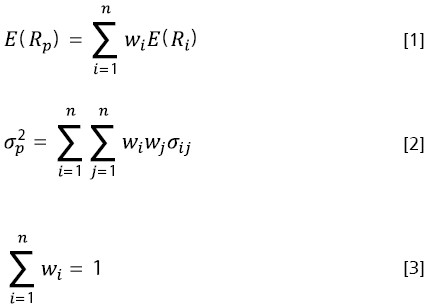Where E(Rp) is the expected rate of return for the portfolio, E(Ri) is the expected rate of return for the stock i, σ2p is the portfolio variance, σij is the covariance between the returns of stocks i and j, and wi (wj) is the proportion of the total budget invested in stock i (j). Thus, to diversify according to one's risk preferences, it is important to take into account the variances of the stocks as well as their covariances.

Therefore, a risk adverse agent would prefer to allocate his resources on an efficient portfolio, i.e., a portfolio in the set of portfolios which have the minimum variance given a certain level of expected return.

When investors include a risk free asset among their alternatives, it is possible, as Tobin (1958) showed, to find an optimal portfolio (in the sense that gives the highest return given the standard deviation) formed of risky assets, which can be combined with the riskless asset in a proportion that depends on the risk preferences of the investor. Mathematically, the proportion of the total budget that one should invest in each of the risky assets to obtain an optimal portfolio can be found maximizing the following expression, also known as the Sharpe ratio: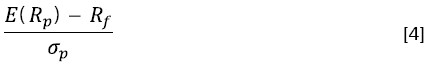Where Rf is the risk-free rate of return. Thus, in this model, the value of the risk premium is the difference between the rate of return for the optimal portfolio of risky assets and the risk-free rate of return (Ibbotson & Goetzmann, 2005). For a market in which all agents take their investment decisions based on expected values and variances3 for the portfolios, and utilize the Markowitz-Tobin model having all of them the same estimates of the inputs needed, Sharpe (1964) and Lintner (1965) developed a model of market equilibrium.

This equilibrium is based, in part, in the idea that the returns for a stock are affected by micro events, i.e., those which affect the company alone, and macro events, e.g., business cycles. Thus, if one makes an ordinary least squares regression of the rates of return for a stock over the rates of return for a market index, then the effects of the macro events on the individual stock can be captured by the beta of this regression.

Additionally, if the market index is an optimal portfolio, then there is a deterministic and linear (cross-sectional) relationship between the expected returns for the stocks in the market and their betas. This model is known as the Capital Asset Pricing Model (CAPM) and establishes that the expected return for any risky asset equals the risk-free rate of return, plus a risk premium: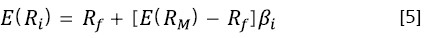In the above equation, E(Ri) is the expected value of a stock i, Rf is the risk free rate, and [E(RM) - Rfβ]i is the risk premium for the stock being considered. In turn, the risk premium comprises the beta factor, which measures the sensitivity of the stock's rate of return to changes in the market return, and the equity risk premium, E(RM) - Rf, which is the premium for the market portfolio. Of course one could expect that the beta factor changes over time with the changes in the general state of the economy. This situation has been studied in papers like Ferson & Schadt (1996) who proposed a formulation in order to estimate a time-varying conditional beta, which is consistent with the CAPM (and the semi-strong form of market efficiency)4.

Since the general state of the economy is captured by the behavior of the market return, then a high beta implies that the stock tends to pay off more when the level of economic activity (including consumption) is high and consequently the marginal utility is relatively low. Therefore, the stock will sell for a relatively low price and have a relatively high average rate of return (Mehra, 2003). This relationship between the equity risk premium and the level of economic activity can also be seen in the Consumption-Based Capital Asset Pricing Model of Breeden (1979) which in turn is a generalization of Merton's (1973) model. Finally, in general terms:

The deus ex machina in asset-pricing theory is that assets are priced in such a way that, ex ante, the loss in marginal utility incurred by sacrificing current consumption and buying an asset at a certain price is equal to the expected gain in marginal utility contingent on the anticipated increase in consumption when the asset pays off in the future (Mehra, 2003, p. 55).

Business cycles refer to the fluctuations of aggregate economic variables about their long-run trend, and there have been important efforts to identify the features of these fluctuations.

Nowadays, to capture the short-run behavior of the level of economic activity, the cyclical components are extracted from the series, finding its long-run trend and then making a differential between the observed value and the trend. Usually this process is done through the mechanical filter of Hodrick-Prescott (1997), but little or nothing has been explored about the Kalman (1960) filter in this field5.

While the first method is the most used, in recent decades has also been one of the most criticized because the exogenous determination of the required smoothing parameter is up to the discretion of the investigator, and the ends of the trends are not correctly defined and lead to a possible spurious behavior in the observed data. Hence, this paper also introduces the Kalman (1960) filter as an alternative to capture long-run trends and thus estimating cycles. This filter is basically an algorithm to process data, which allows obtaining long-run trends for non-stationary series6.

Following Misas & López (1998, p. 5) this filter performs a much more stylized decomposition than the previous methods mentioned, since the cyclic part of the series is explicitly specified, and the trend is more related to the shocks of the series, i.e. its potential component. On the other hand, allows, unlike other methods:

The representation of a state-space flexible enough to incorporate a structure with sufficient information, but that maintains its parsimony. In addition, this methodology can generate confidence intervals and projections out of sample directly from the estimates, a result that is very attractive when formulating policies (Gallego & Johnson, 2001, p. 31).

It should be noted that the results should differ in their quantitative values because of the different methods utilized, but the results should be qualitatively similar.

The mechanical filter of Hodrick- Prescott (FHP)

The conceptual framework of this filter consists in that a series Yt, equals the sum of its growth and its cyclical components. Thus the values above or below the trend are: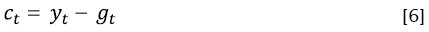Where gt and Ct are the growth and the cyclical components respectively. If the average of Ct is approximately zero for long time periods, then the growth component can be found solving the following programming problem (Hodrick & Prescott, 1997, p. 3):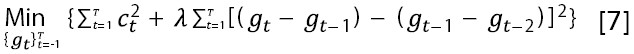Where λ is the parameter that penalizes deviations around the trend component and hence the higher its value, the smoother the series found7. Thus, periods in which the difference between the observed value of economic activity and its trend is greater than zero Ct > 0 correspond to times of high levels of economic activity (expansion) and periods where the difference is less than zero (Ct > 0), reflect periods of low economic activity (recession).

The Kalman filter (FK)

To start with the Kalman filter, it is necessary to set the state-space model that estimates the trend:

The filter assumes a vector of state variables βt that define a system at a given time, which are not observable directly or immediately. The only thing known is the scalar or vector Yt , which is a linear combination of state variables plus an error term that meets the classical assumptions. The equation is called the measurement equation or the observations equation. In these terms, the measurement and state equations, both establish the formulation of the state-space model of the Kalman filter (Montenegro, 2005, p. 4).

In this case it is assumed that the trend follows a local linear trend model defined by the following state variables: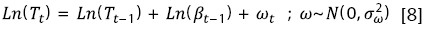The above equation shows the structure of the measurement equation. For the equation of state, it is assumed that βt follows an AR (1) process. Thus: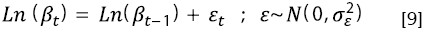Finally, the process is estimated through maximum likelihood, utilizing for this case, the optimization algorithm of Marquardt. Regardless of the method used to find the trend, the cycle is obtained from the difference between the observed behavior of the series and this trend, resulting in deviations from the former relative to the latter. To understand how to extract the trend, there must be distinguished four components (unobservable) in time series: the trend (YT ), the cycle (Ct ), seasonality (St), and an irregular or random component (Ut):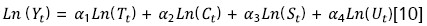Taking expected values on both sides of the equation gives: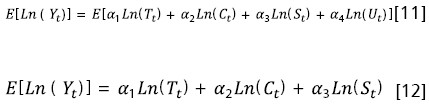In this sense, the expected cycle of any time series is obtained from the difference between the value of the series and its trend: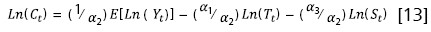Finally, removing the seasonal component according to the X12 method, the cycle is determined by: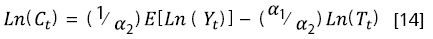EMPIRICAL ANTECEDENTS

The relationship between risk premiums and economic activity has been studied intensively at least for the last two decades, and most of the international evidence has shown a countercyclical pattern as documented by Gómez & Astaiza (2013). For example, Ferson & Harvey (1991) showed graphically that the expected equity risk premium rose during the economic contractions in the United States. This was found using a market-weighted stock index, the rate of return for one-month T-Bills and information of the National Bureau of Economic Research for the economic cycles. Also, there is supporting evidence about the existence of a relationship between the ex-post equity risk premium in emerging markets and global business cycles, as reported by Salomons & Grootveld (2003). In a similar way, Lettau, Ludvigson & Wachter (2008) found evidence of a strong correlation between the stock prices in the United States and macroeconomic volatility, measured through the GDP and the per capita personal consumption. Furthermore, Lustig & Verdelhan (2012) reported that the average excess return was 11,3 % during the postwar recessions, which was greater than the 5,3 % registered during expansions in the United States.

At the national level, one of the first authors who analyzed the features of the economic cycles through the Hodrick-Prescott filter is Zucardi (2002). The aim of his research was to establish the relationship between the national business cycles and the regional business cycles during the years 1986 and 2000, using cities as the basic area units. It was found that Bogotá, Medellín, Cali and Bucaramanga, in this order, were more sensitive to the shocks that affected the whole country than to those which affected the regions in which they are inscribed to, and therefore, they had a stronger relationship with the national cycles. At the same time, Pasto, Barranquilla and Manizales were more sensitive to the shocks that affected their own regions.

With regard to the applications of the econometric filters to obtain the cycles of economic series, it was found a study made by Gómez (2011) whose objective was to investigate the cyclic interdependence of the GDP of Cauca with its neighboring administrative regions or departamentos. The Kalman and Hodrick-Prescott filters were used in order to estimate the regional business cycles, and VAR systems with impulse-response analyses were implemented with the purpose of determining the influence of the cycle of Cauca and exogenous events, on the rest of the region and vice versa. The findings showed that for the two methodologies, the cycle of Cauca had a stronger relationship with Nariño than with Huila, Valle or Tolima, and that the exogenous events showed a much more volatile impacts for the Kalman filter than for the Hodrick-Prescott one.

In the field of finance and the behavior of the Colombian GDP, Astaiza (2012) exposed a theoretical revision of the equity risk premium as well as a method to estimate its historical values for Colombia, and its comparison with the GDP behavior. The results, among others, revealed the existence of a negative (Pearson) correlation between the historic ERP and the lagged series of the GDP growth, suggesting that during the times of low or negative levels of economic growth, agents perceived less certainty about the future and transferred their risk perception towards the ERP.

Finally, in a first effort to show, at the national level, the relationship between the ex-post equity risk premium and the business cycles in Colombia, Gómez & Astaiza (2013), under the methodology of the mechanical Hodrick-Prescott filter, showed that the fluctuations of the ex-post equity risk premium followed a countercyclical pattern, which is in accordance with the research conducted so far, for the United States and emerging markets, but with a non-contemporary relationship with the private consumption. Additionally, they found that in the last decade the equity risk premium in Colombia followed an autoregressive moving average process ARMA , which did not change for at least two consecutive quarters and whose behavior was generated by exogenous events at the level of the domestic economic activity occurred in recent past periods.

DESCRIPTION OF DATA

There are two main variables in this study: the observed economic activity and the ex-post equity risk premium (ERP), all of which have monthly observations from February 2008 to February 2014, so 73 observations are included. At the same time, the ex-post ERP is the difference between the return on equity and the risk free rate of return.

The changes in the Colombian economic activity were captured using two indexes: the Sales Index with coffee threshing (SI) from the Monthly Manufacturing Sample, and the Retail Trade Index (RTI) without fuel from the Monthly Retail Trade Sample, both conducted by the Departamento Administrativo Nacional de Estadística (DANE). Since they are expressed in a monthly basis, the series show seasonal components basically in the month of December. The seasonal adjustment was done using the X-12 method for monthly data, implemented by the U.S. Census Bureau. Following Cortez (2008), this method "is based on moving averages which are sustained on the time or frequency dominance and achieves the seasonal adjustment with the development of a system of factors that explain the seasonal variation of the series" (p. 6).

The monthly risk free rate was calculated based upon the Central Bank's minimum rate for auctions on one-day monetary expansion operations8. There are three main reasons for this. In the first place, there were not observations of the government zero-coupon bonds for several periods. Moreover, there are not one-month securities issued by the Colombian government. Second, as a proxy for the rates of return of investments similar to securities with no default risk, no reinvestment risk and maturities of less than one year, empirical studies in Colombia usually utilize the Central Bank's policy rate (Jara, 2006; Martínez & Murcia, 2007; García & Moreno, 2011; Gómez & Astaiza, 2013). Third, since the variance of the Central Bank's rate in each of the months in the sample was approximately zero9, this rate meets the statistical properties of a risk free rate of return10.

Thus, the risk free rate for each month was estimated converting the observations of each day to logarithmic terms and then transforming them to their monthly equivalent. Subsequently, the average for each month was calculated.

For the returns on equity, there were used the monthly values of the Colombian market index Colcap11, from which the logarithmic rates of return were calculated for each month. Then, the ex-post ERP was calculated subtracting the risk free rate from the rate of return on the Colcap. The distributional characteristics of the Colombian ERP are shown in tabla 1: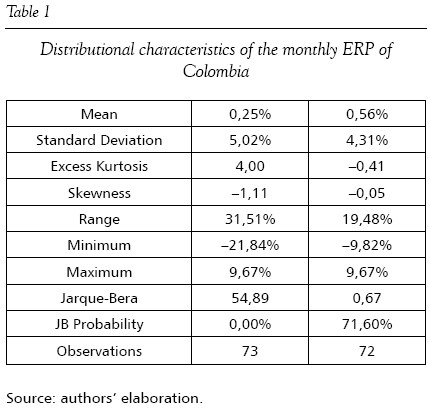As can be seen, the expected value of the continuous ERP of the sample was positive and its maximum monthly value was 9,7 % which appeared in May 2009. It also can be seen that the minimum value of the sample, -21,84 %, is an outlier which corresponded to the observed ERP of October 2008. If this outlier is taken out from the series then the sample with 72 observations has a minimum with an absolute value of 9,82 %, very close to the maximum, and a higher expected value of 0,56 %, which is more than twice the expected ERP of the total sample.

Also, the complete sample has a higher kurtosis compared to that of a normal distribution and a negative skewness. But if the outlier is taken out from the sample and the Jarque & Bera (1980) test is applied, then it is not possible to reject that the Colombian logarithmic ERP had a normal distribution between February 2008 to February 2014. In effect, this is because the P-value exceeds the 5 % statistical significance. Although it is not shown here, the discrete ERP was also normally distributed12, and consequently it was consistent with standard portfolio theory in the sense that the mean and the variance is all what matters to investors. In addition, the observations used in Gómez & Astaiza (2013) also followed a normal distribution during the period from 2002 to 2007 according to the Jarque & Bera test (1980).

RESULTS OF THE CYCLES THOROUGH FHP AND FK

Initially to follow the Box-Jenkins methodology, the series RTI and SI were taken in logarithms to reduce its variance. Secondly, and to be consistent with the requirements of equations  and , a log-additive decomposition method was applied when the series were seasonally adjusted through the X-12 method. The results (not exposed here) show that the correlation between the observed and the seasonally adjusted series have a relationship of 0,68 for the first one and 0,88 for the second one, results that are statistically significant13.

Finally, the series under analysis evince to be integrated of order one [I(1)], through the Augmented Dickey-Fuller (ADF) unit root test14. So, the Kalman filter can be applied without any problem given that the series under analysis are non-stationary. In precise terms, the results of the filter show a good fit, since the coefficients have p-values equal to zero, and the same happens for the state vectors versus the root mean square error (RMSE). Also, the information criteria show minimum values, reinforcing this appreciation 15 (table 2).

In the Hodrick-Prescott case the trend is determined directly without estimating any model. It must be remembered that proponents of the filter themselves recommended that the smoothing parameter is λ = 14400 for monthly data, λ = 1600 for quarterly data, and λ = 100 for annual data. In the present study the first recommendation was followed because of the monthly nature of the data. Therefore, in graphic terms the Kalman and Hodrick-Prescott trends are the following:

The figures above evince that the trend of Kalman (FK) is better adjusted to the volatility of the series compared to the trend extracted with Hodrick-Prescott (FHP). The correlation between the trend and the observed series is 0,94 for the first and 0,99 for the second case. The trend of FHP, overestimates the observed data of RTI during all 2009, and throughout 2009 and 2010 for the SI series. In the extreme years it tends to have a better fit, especially in the case of RTI. For Kalman, the values are much more consistent with observed reality, and there is undervaluation or overvaluation only when both series take extreme values, especially in the case of SI. In these terms, according to equation , the cycles of both series by the two methods look as follows.

As can be seen, the cycles replicate what was previously exposed about the undervaluation or overvaluation of the observed data. In both cases these scenarios were present for the intermediate years (2009 to 2011) but not for the extreme ones (2008 and 2012-2014). Finally, it is worth noting that there is no need to extract a cycle from the risk premium series (EPR), because as a variable in first differences, it is already stationary by definition and therefore has no trend. To be redundant, the ERP is clearly a short-run variable and not a long-run one. Its behavior compared with the cyclical variables RTI and SI, in the FHP and FK scenarios are shown in the following Graph16.

Graph 1

Graph 2

Graph 3

Graph 4

Graph 5

Graph 6

In the FHP case, the cycles show that the equity risk premium (ERP), keeps a counter-cyclical relationship with both the Sales Index with coffee threshing (SI) and the Retail Trade Index (RTI) without fuel because the correlations are equal to -0,24 and -0,30, respectively. In the FK case, the situation is the same, as ERP, SI and RTI have a countercyclical relationship equal to -0,11 and -0,17, respectively. Therefore, for both scenarios, times of high risk premiums compaginate with low levels of SI and RTI, and vice versa. As correlation does not imply causation, it is necessary to build a model that captures the behavior of these variables in the short run.

ECONOMETRIC MODELS

Once established the cyclical behavior of SI and RTI, a model that relates the equity risk premium with the cyclical behavior of SI and RTI in the FHP and FK scenarios, is proposed. Then: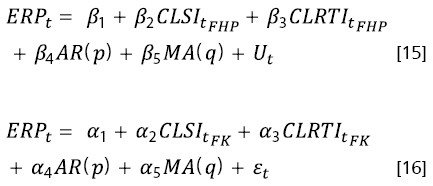In equation (11) ERPt corresponds to the ex-post equity risk premium, CLSItFHP denotes the cycle in logarithms of SI, and CLRTItFHP shows the cycle in logarithms of RTI, both under the Hodrick-Prescott scenario. In equation  ERPt is again the equity risk premium, CLSItFK denotes the cycle in logarithms of SI, and CLRTItFK shows the cycle in logarithms of RTI, now both under the Kalman scenario. The autoregressive variable AR (p) is included in both models in order to avoid potential problems of autocorrelation, and to raise the possibility that the ERP in such short periods (months) cannot change in a rapid way, by which the value today of this variable is likely to depend on what happened in previous months. Also, because stock variables have a very volatile behavior, especially in the presence of unforeseen events, a moving average MA(q) is included. Finally, both Ut and εt are random variables that meet the classical assumptions of zero mean, constant variance and covariances equal to zero. They denote variables that affect the ERP but that have not been explicitly taken into account in the models17.

It should be noted that the cycles or the variables in differences are stationary by definition, i.e. the order of integration is zero (I). Therefore, a unit root or cointegration analysis for the series is not needed, ensuring that the estimates of both models are not spurious.

It is expected that the relationship between the equity risk premium and the cycles of the Sales Index with coffee threshing and the Retail Trade Index (RTI) without fuel, is negative, which would be consistent with the investment-consumption model in the sense that:

When business conditions are poor, income is low and expected returns on bonds and stocks must be high to induce substitution from consumption to investment. When times are good and income is high the market clears at lower levels of expected returns (Fama & French, 1989, p. 48).

Add to this, a negative relationship between the Colombian ex-post ERP and the economic activity was found in Gómez & Astaiza (2013). With regard to the autoregressive variable AR(1), its sign is not clear a priori given that the changes in the ERP could be transferred or not to the next period, depending on the situation. Similarly, with respect to the process MA(1), its random nature impedes to know ex ante its sign. In order to capture in a better form the variance of the ERP, there were explored different functional forms which allowed improving their fit, introducing intertemporal phenomena. The overall results are shown below (tables 3 - 4).

The estimates in both scenarios show similar results. The initial explored functional forms show low individual and overall statistical significances, although the p-values of the F-tests are below than 5 %. Concerning the signs, all of them are as expected, i.e. negative under the hypothesis of counter-cyclical relationships. The functional forms 4 and 5 show the best goodness of fit, especially the last. In these, the considered variables have statistical significances below 10 %, and the R-squared reaches 27 % for FHP and 31 % for FK, with p-values equal to zero for the F-tests. In neither case there is intercept, because the ERP is captured in differences and consequently starts very close to zero, as shown in the figures above18.

Under such circumstances, the estimates of the functional form number five, show that the relationship between the ERP and the variables of economic activity, i.e. SI and RTI, is inverse or counter- cyclical, but not contemporary. In other words, if the values of SI or RTI were relatively high two months ago, the current equity risk premium is relatively low and vice versa, ceteris paribus. Regarding the autoregressive process AR(2), it is evinced that the ERP does not change very rapidly from one month to another, i.e. it does not fluctuate widely, at least not for two consecutive months. Finally, the process MA(2) indicates that the ERP depends on external random shocks in a non contemporary manner of up to two months back. The origin of these random shocks is unknown but they could come from causes exogenous from the economy such as politics or aspects of social character.

In comparative terms, the functional form number five in the Kalman case has a better fit since its R-squared is greater than the one in the Hodrick- Prescott scenario, considering in both cases exactly the same explanatory variables.

CONCLUSIONS

In this study it has been found that the methodology of the Kalman filter can be applied to financial series as well as to economic series, with notorious statistical advantages compared with the commonly used methodologies (Hodrick-Prescott filter). Indeed, its application generates a better fit since it provides a more robust and accurate estimation of the long term behavior of the series and consequently, it does not overestimates or underestimates the behavior of the cycle (short-term), situation that does occur when the other method is used. This finding constitutes a contribution to the studies related to the analysis of the ERP and its relationship with the business cycles.

Also, this document confirms the advantages expressed by Misas & López (1998), as well as by Gallego & Johnson (2001), of the Kalman filter against the Hodrick-Prescott one, at least in regard to the goodness of fit of the long-run trends that both filters generate. Indeed, the estimated correlation between the observed series of the Retail Trade Index and its trend, reach 0,99 in the Kalman scenario while in Hodrick-Prescott amounts to 0,97. In the case of the series of the Sales Index with coffee threshing, the Kalman filter gives a correlation of 0,94 and the Hodrick-Prescott filter amounts 0,89. In other words, the Kalman filter generates a better fit independently of the series. Thus, the use of the Kalman filter, and not the Hodrick-Prescott filter, is recommended to build the cycles of the variables that attempt to capture the relationship between the equity risk premium and the level of economic activity.

The Equity Risk Premium had an outlier and was normally distributed when it was taken out of the sample, presenting a maximum of 9,67 %, a minimum of -9.82 %, an unconditional mean of 0,56 %, and a standard deviation of 4,31 %. Also, it did not change rapidly from one month to another, as the AR (2) variable shows, and depended on random shocks as can be seen from the MA (2) explanatory variable.

Finally, the relationship between the variables of economic activity and the ERP was countercyclical but not contemporary which is similar to what was found in Gómez & Astaiza (2013). Specifically, when the values of SI or RTI lagged by two months were relatively high, the current ERP was relatively low and vice versa, ceteris paribus.

NOTES

1 There can be identified four perspectives of the ERP: historic or ex-post, expected, required and implicit (Fernández, 2009). Volver

2 The ERP, which is a variation rate, and its cycle were essentially the same since they did not have a trend. Volver

3 Agents can invest their money on the basis of the expected values and variances if the rates of return of the portfolios are normally distributed, since a normal distribution can be fully described by its mean and variance. Alternatively, if investors have a utility function that is related to their wealth in a quadratic form, they would only care about expected values and variances. If a utility function such as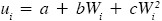is included in the expression of the expected utility Σ uihi then it is possible to observe that E(u) = a + bE(W) + cVar (W)Σ, where Wi is the i-th level of wealth, hi is the probability of occurrence of the i-th level of utility, and the coefficients b and c are positive and negative respectively. Volver

4 In the applications of the CAPM theory, other variables besides the market returns are included like those of the three-factor model of Fama & French (1993), and the four-factor model of Carhart (1997). Volver

5 There are various other ways to find the trend of a series besides the abovementioned such as the bands filter of Baxter & King, the linear-quadratic-cubic adjustment of the trend, the PAT (Phase Average Trend) and the method and Loess Fit. Volver

6 It is also useful for estimating autoregressive models, or models with unobservable components specified in the state-space form. Volver

7 Following Mise, Kim & Newbold (2005, p. 55), an appropriate value for λ is 14400 for monthly data. Volver

9 To be more precise, in 72 of the 73 months included, the variance in annual equivalents was less than 0,001 % and in 62 of them, it was smaller than 0,0001 %. The greatest variance was registered in march 2008, when its value was 0,002 %. Volver

10 If its variance equals 0, then its covariance with the returns on any other risky investments is also zero. Volver

11 The series of the Colcap can be downloaded from www.bvc.com.co Volver

12 The skewness of the discrete sample is 0,06 and the Jarque Bera p-value 73,66 %. Note that the ERP of each month was estimated using logarithmic returns, as in the mathematical approach developed in Mehra (2003). Specifically, the ERP was estimated as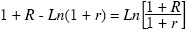. The excess of wealth earned, per unit of money, in the stock market over the wealth earned by investing in the risk free asset is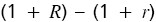where R is the stock market discrete return and r is the discrete risk free rate of return. Hence, the effective or discrete ERP is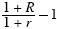which for small values of r is approximately R - r as is the case of this study. Since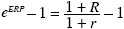, then the continuous ERP equals Ln(Efective ERP + 1) = ERP. Volver

13 To formalize this result, a statistical test of simple correlations was applied, where the null hypothesis HO (no correlation) faces the alternative hypothesis H1 (correlation exists). The t-student distribution was chosen to test the null hypothesis and the test statistic (tC):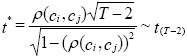Where t * is the test statistic (tC); ρ(ci,cj) represents the correlation coefficient between the variables i and j, and T is the number of sample data. The test statistic follows a t-student distribution with (T-k) degrees of freedom, and an α level of statistical significance. The result supports the alternative hypothesis of correlation between the two filters. Volver

14 These results are shown in appendices (1) and 2). Volver

15 Specific results are given in appendices 3) and 4). Volver

16 The graph 5 shows the normalized series because if the observed values were taken, its metric would impede to compare them. It should be noted therefore that the measurement is made now in standard deviations. Volver

17 The causal relationship of the model may not be necessarily contemporary, in other words, the current ERP could be influenced by previous behaviors of the considered variables. Volver

18 In this type of models where all variables are cyclical, i.e. no trends are present and their behavior is erratic or uneven, their fit regularly tends to be poor, as shown by the results. Volver

REFERENCES

1. Astaiza, J. (2012). Prima por riesgo en el mercado colombiano. Valores, Finanzas y Administración, 1(1), 16-23.         [ Links ]

2. Breeden, D. (1979). An intertemporal asset pricing model with stochastic consumption and investment. Journal of Fianancial Economics, 7, 265-296.         [ Links ]

3. Carhart, M. (1997). On persistence in mutual fund performance. Journal of Finance, 52, 57-82.         [ Links ]

4. Cortez, J. (2008). Desestacionalización X12 ARIMA con efecto calendario. Series Instituto Nacional de Estadística, 12, 1-51.         [ Links ]

5. Fama, E. & French, K. (1989). Business conditions and expected returns on stocks and bonds. Journal of Financial Economics, 25(1), 23-49.         [ Links ]

7. Ferson, E. & Harvey, C. (1991). The variation of economic risk premiums. Journal of Political Economy, 99(2), 385-415.         [ Links ]

8. Ferson, W. E. & Schadt, W. (1996). Measuring fund strategy and performance in changing economic conditions. Journal of Finance, 51(2), 425-461.         [ Links ]

9. Gallego, F. & Johnson, C. (2001). Teorías y métodos de medición del producto de tendencia: una aplicación al caso de Chile. Economía Chilena, 4(2), 27-58.         [ Links ]

10. García, C. & Moreno, J. (2011). Optimización de portafolios de pensiones en Colombia: el esquema de multifondos, 2003-2010. Ecos de Economía, 15(33), 139-183.         [ Links ]

11. Gómez, M. (2011). Análisis de la interdependencia de los ciclos económicos del Cauca y el suroccidente colombiano: una aproximación econométrica desde los filtros de Kalman y Hodrick-Prescott. Estudios Gerenciales, 27(121), 115-141.         [ Links ]

12. Gómez, A. M. & Astaiza, J. G. (2013). Ciclo económico y prima por riesgo en el mercado accionario colombiano. Ecos de Economía, 37, 109-124.         [ Links ]

13. Hodrick, R. & Prescott, E. (1997). Postwar U.S. business cycles: an empirical investigation. Journal of Money, Credit and Banking, 29(1), 1-16.         [ Links ]

14. Ibbotson, R. & Goetzmann, W. (2005). History and the Equity Risk Premium. Yale ICF Working Paper, 05-04. Retrieved from SSRN: http://ssrn.com/abstract=702341        [ Links ]

16. Jarque, C. & Bera, A. (1987). A test for normality of observations and regression residuals. International Statistical Riview, 55, 163-172.         [ Links ]

17. Kalman, R. E. (1960). A new approach to linear filtering and problems. Journal of Basic Engineering, 82, 35-45.         [ Links ]

18. Lettau, M., Ludvigson, S. & Wachter, J. (2008). The declining equity risk premium: what role does macroeconomic risk play? Review of Financial Studies, 21(4), 1653-1687.         [ Links ]

19. Lintner, J. (1965). Security prices, risk, and maximal gains from diversification. The Journal of Finance, 20(4), 587-675.         [ Links ]

20. Lustig, H. & Verdelhan, A. (2012). Business cycle variation in the risk-return trade off. Journal of Monetary Economics, 59, 535 - 549.         [ Links ]

21. Markowitz, H. (1952). Portfolio selection. The Journal of Finance, 7(1), 77-91.         [ Links ]

22. Martínez, O. & Murcia, A. (2007). Desempeño financiero de los fondos de pensiones obligatorias en Colombia. In Banco de la República, Reporte de estabilidad financiera, (pp. 75-90). Bogotá: Author. Retrieved from http://www.banrep.gov.co/publicaciones/pub_es_fin.htm2007        [ Links ]

23. Merton, R. C. (1973). An intertemporal capital asset pricing model. Econometrica, 41, 867-887.         [ Links ]

24. Mehra, R. (2003). The equity premium: Why is it a puzzle? Financial Analysts Journal, 59(1), 54-69.         [ Links ]

25. Mill, J. S. (1848). Principles of political economy with some of their applications to social philosophy. Retrieved from http://www.econlib.org/library/Mill/mlP30.html        [ Links ]

26. Misas, M. & López, E. (1998). El producto potencial en Colombia: una estimación bajo un VAR estructural. Borradores Semanales de Economía, 94, 1-40.         [ Links ]

27. Mise, E., Kim, T., & Newbold, P. (2005). On suboptimality of the hodrick-prescott filter at time series endpoints. Journal of Macroeconomics, 27(1), 53-67        [ Links ]

28. Montenegro, A. (2005). Introducción al filtro de Kalman. Documentos de Economía, 18, 1-23.         [ Links ]

29. Salomons, R. & Grootveld, H. (2003). The equity risk premium: emerging vs. developed markets. Emerging Markets Review, 4(2), 121-144.         [ Links ]

30. Sharpe, W. F. (1964). Capital asset prices: a theory of market equilibrium under conditions of risk. The Journal of Finance, 19(3), 425-442.         [ Links ]

31. Tobin, J. (1958). Liquidity preference as behavior towards risk. The Review of Economic Studies, 67, 65-86.         [ Links ]

32. Zuccardi, I. (2002), Los ciclos económicos en Colombia, 1986-2000. Documentos de Trabajo Sobre Economía Regional, 25, 1-50.         [ Links ]

APPENDICES

Volver

Volver

Volver

Volver